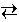# Chemical Equilibria

## Basic Concepts

Consider the following gas-phase chemical reaction:       H2 + I22 HI

Imagine a small mixing chamber into which gaseous H2 and I2 are rapidly introduced. Initially there is no HI, H2 is present at 0.20 M concentration, and I2 is present at 0.024 M concentration. As time goes by, however, the hydrogen and iodine react to form HI, as shown in the simulation below.
Click on the Start Simulation button and observe how the concentrations of the three compounds change with time.

As the reaction progresses, the concentrations of iodine and hydrogen decrease as they are consumed while the concentration of hydrogen iodide increases as it is formed. Eventually, however, the concentrations of all three species reach constant values. This behavior is a results of the back reaction in which hydrogen iodide reacts to form iodine and hydrogen. Initially there is no hydrogen iodide, so the back reaction cannot occur. As hydrogen iodide accumulates, the back reaction becomes significant.

Eventually the rate at which iodine is being consumed by the forward reaction is perfectly balanced by the rate at which it is being produced by the back reaction. The same is true for hydrogen and hydrogen iodide. When the rates of forward and reverse reactions are perfectly balanced in this way, the reaction is said to be at equilibrium.

### The Law of Mass Action

When a chemical reaction reaches equilibrium, the concentrations of the reactants and products obey the Law of Mass Action.

For the generic chemical reaction

a A + b Bc C + d D

the Law of Mass Action is

 KC = [C]c [D]d[A]a [B]b

The uppercase letters represent chemical species while the lowercase letters represent the corresponding stoichiometric coefficients. The notation [A] represents the molar concentration of species A.

In this expression, the numerator contains the concentration of each product raised to the power of its stoichiometric coefficient. The denominator contains the corresponding expression for the reactants.

If the species are gases, an entirely analogous expression may be written using partial pressures instead of molar concentrations.

 KP = PCc PDdPAa PBb

The equilibrium constant KC applies to an equilibrium expression written in terms of molar concentrations, and the equilibrium constant KP applies to an equilibrium expression written in terms of partial pressures (with units of atm).

One can convert between KC and KP using the ideal gas law: P = R T C

The equilibrium constant characterizes the "position" of the equilibrium. The larger the equilibrium constant, the more the equilibrium favors products; the smaller the equilibrium constant, the more the equilibrium favors reactants. In an ideal system, the equilibrium constant depends only upon the temperature of the system.

Note: Only species that are gases or solutes appear in the equilibrium expression. Pure solids and pure liquids do not appear in the expression.

Equilibrium ConstantChemical Equilibria Home PageVirtual Chemistry Home Page

BasicConcepts.html version 2.2